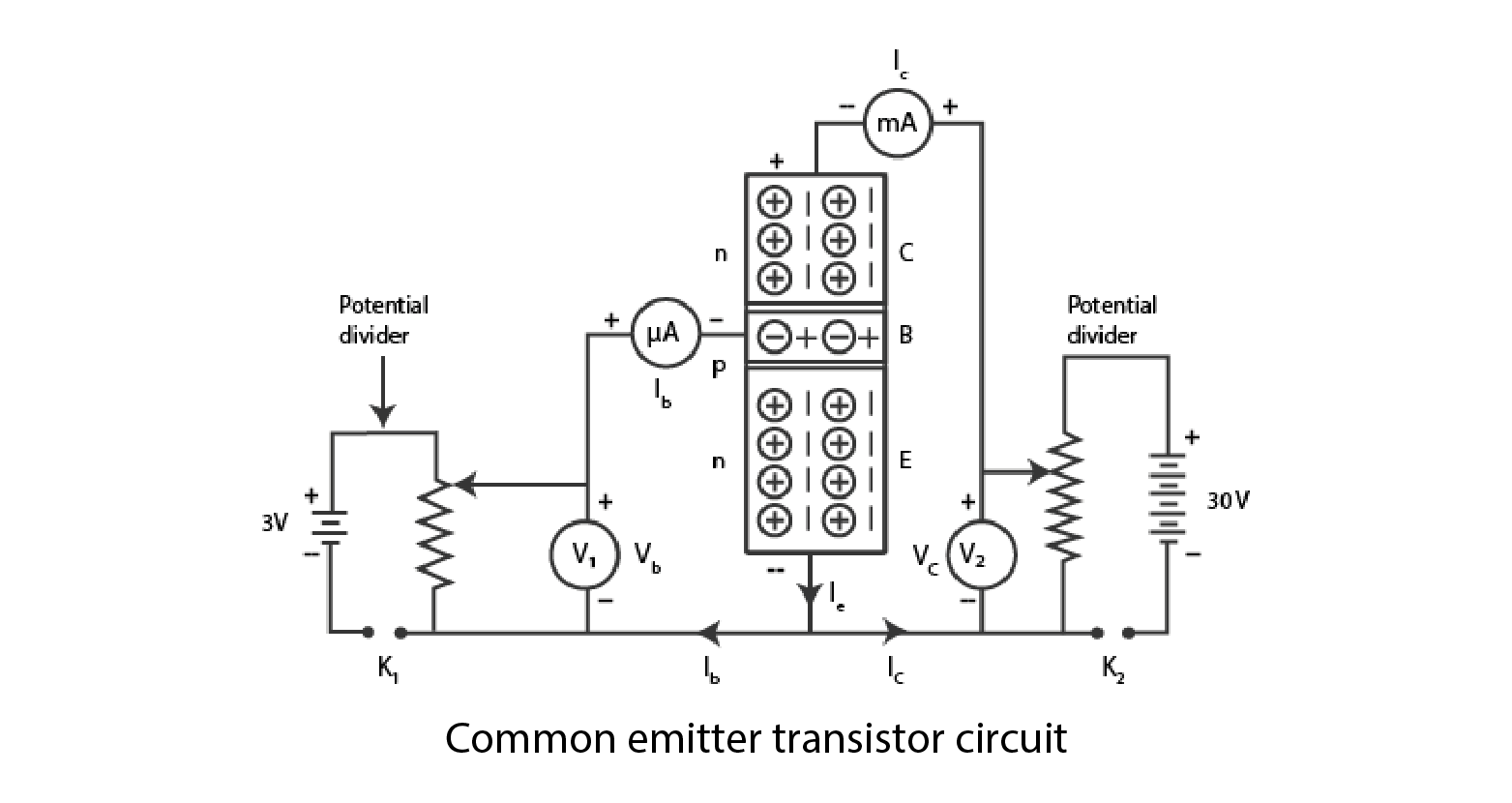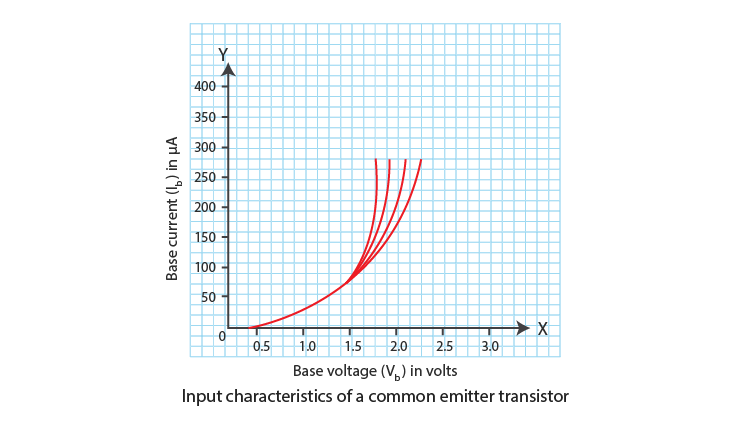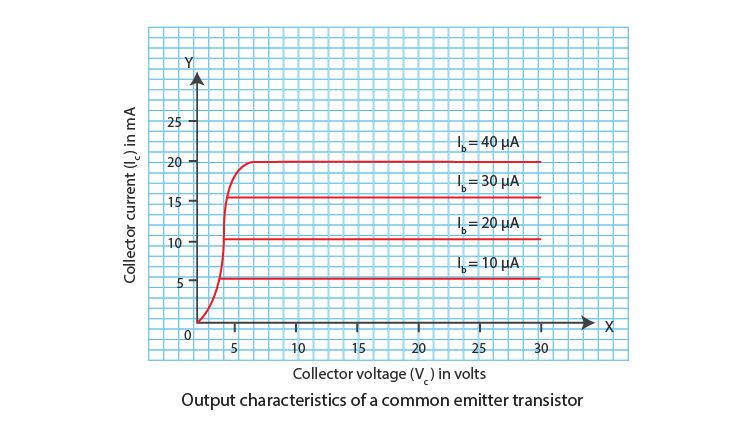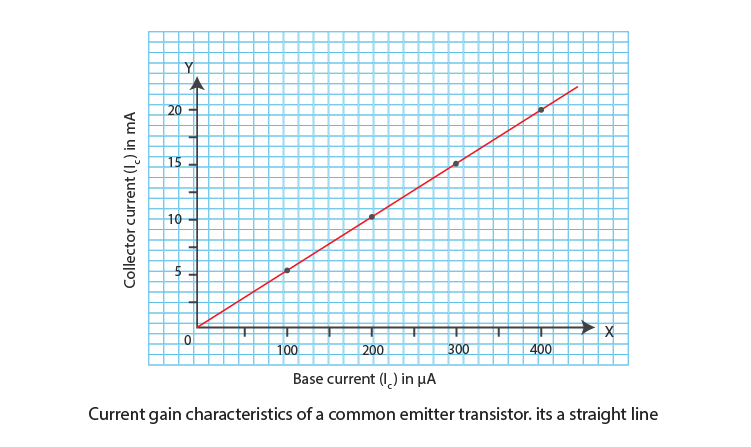# To study the characteristics of a common emitter npn or pnp transistor and to find out the values of current and voltage gain

## Aim

To study the characteristics of a common emitter npn or pnp transistor and to find out the values of current and voltage gains.

## Materials Required

1. An n-p-n transistor
2. A 3-volt battery
3. A 30-volt battery
4. Two high resistance rheostats
5. One 0-3 volt voltmeter
6. One 0-30 volt voltmeter
7. One 0-50 μA micro-ammeter
8. One 0-50 mA milli-ammeter
9. Two one way keys
10. Connecting wires

## Theory

### What is input resistance?

Input resistance (RI) is defined as the resistance offered by the collector junction and has a very small resistance due to forward biasing. It is given as:

 $R_{I}=\frac{\Delta V_{b}}{\Delta I_{b}}$

### What is output resistance?

Output resistance (RO) is defined as the resistance offered by the collector junction and has a high value due to reverse biasing. It is given as:

 $R_{O}=\frac{\Delta V_{c}}{\Delta I_{c}}$

### What is current gain?

Current gain (β) is defined as the ratio of change in collector current to the change in base current. It is given as:

 $\beta =\frac{\Delta I_{c}}{\Delta I_{b}}$

## Diagram## Procedure

1. The experimental setup should be according to the diagram.
2. All the connections should be neat, clean and tight.
3. The least count and zero error of voltmeters and ammeters should be noted.
4. Before inserting the keys, the voltmeter readings in V1 and V2 should be zero.

For input characteristics

1. To read the base voltage (Vb) and base current (Ib) from μA, a forward bias voltage needs to be applied on the base junction.
2. Vb should be increased till Ib increases suddenly and for every value of Ib, Vb should be noted.
3. Repeat steps 5 and 6 by making the collector voltage Vc = 10 V.
4. With Vc = 20 V and 30 V, repeat step 7.
5. Make all the readings zero.

For output characteristics

1. Note the collector current Ic by adjusting collector voltage Vc zero, and base current Ib = 10 μA by adjusting base voltage Vb.
2. To note the collector current, make the collector voltage 10V, 20V and 30V.
3. With base current Ib=20μA, 30μA and 40μA, repeat steps 10 and 11.
4. Record the observations.

## Observations

Least count of the voltmeter, V1 = …..V

Zero error of voltmeter, V1 = …….V

Least count of the voltmeter, V2 = …..V

Zero error of voltmeter, V2 = …….V

Least count of milli-ammeter = ……..mA

Zero error of milli-ammeter = …….mA

Least count of micro-ammeter = ……..μA

Zero error of micro-ammeter = …….μA

Table for base voltage and base current

 Sl. no Base voltage (Vb) in V Base current (Ib) for collector voltage Vc= 0V in μA Vc= 10V in μA Vc= 20V in μA Vc= 30V in μA

Table for collector voltage and collector current

 Sl.no Collector voltage (Vc) in V Collector current (Ic) for base current Ib = 10μA in mA Ib = 2 0μA in mA Ib = 30μA in mA Ib = 40μA in mA

## Calculations

1. Calculation for input resistance (RI)

Plot graph between base voltage Vb from table-1 and base current Ib from table-2 for zero collector voltage Vc. Vb is on the x-axis and Ib is the y-axis. The graph obtained is called input characteristics of the transistor. The slope gives the value of $\frac{\Delta I_{b}}{\Delta V_{b}}$ which is the reciprocal of $\frac{\Delta V_{b}}{\Delta I_{b}}$2. Calculation for output resistance (RO)

Plot graph between collector voltage Vc and collector current Ic from table-2 for 10 μA. Vc is taken on x-axis and Ic on the y-axis. The graph obtained is called output characteristics of the transistor. The slope gives the value of $\frac{\Delta I_{c}}{\Delta V_{c}}$ which is the reciprocal of $\frac{\Delta V_{c}}{\Delta I_{c}}$3. Calculation for current gain (β)

Plot graph between base current Ib and its corresponding collector current Ic from table-2 for 30V collector voltage Vc. Ib is taken on x-axis and Ic on the y-axis. The graph obtained will be a straight line and is called the current gain characteristic of the common emitter transistor. The slope of the straight line gives the value of $\frac{\Delta I_{c}}{\Delta I_{b}}$ which is equal to β.4. Calculation for voltage gain Av

$A_{v}=\beta *\frac{R_{0}}{R_{1}}$

## Result

For the given common emitter transistor,

Current gain, β = …….

Voltage gain, Av = …….

## Precautions

1. The connections should be neat, clean and tight.
2. Key should be used when the circuit is being used.
3. Beyond breakdown, forward bias voltage should not be applied.
4. Beyond breakdown, reverse bias voltage should not be applied.

## Sources Of Error

Faulty junction diode might be supplied.

## Viva Questions

Q1. What is semiconductor junction transistor?

Ans: A semiconductor junction transistor has three sections and they are:

• Emitter (E)
• Base (B)
• Collector (C)

Q2. How are the three sections arranged in a transistor?

Ans: A thin layer of extrinsic semiconductor is used in the base and on the other two sections the second type of extrinsic semiconductor is used.

Q3. What are the different ways to use a transistor?

Ans: Following are the three different ways in which a transistor can be used:

• Common base circuit
• Common collector circuit
• Common emitter circuit

Q4. What is the importance of the input characteristics?

Ans: The importance of the input characteristics is that it helps in calculating the input resistance of the transistor.

 $R_{I}=\frac{\Delta V_{b}}{\Delta I_{b}}$

Q5. What is the importance of the output characteristics?

Ans: The importance of the output characteristics is that it helps in calculating the output of the transistor.

 $R_{O}=\frac{\Delta V_{c}}{\Delta I_{c}}$